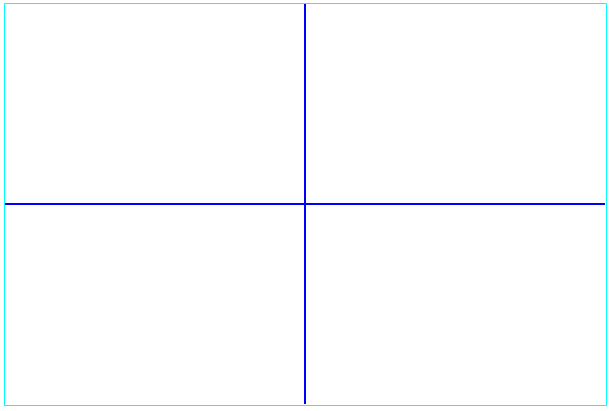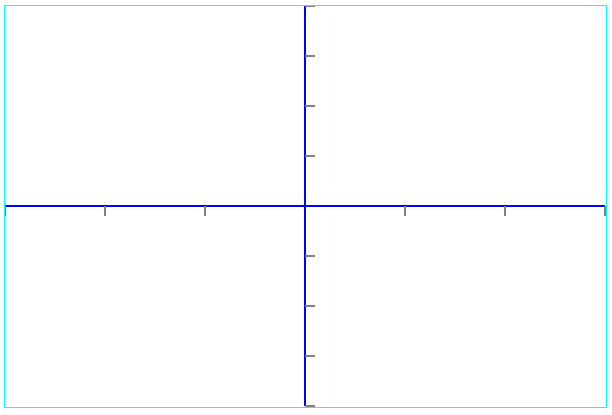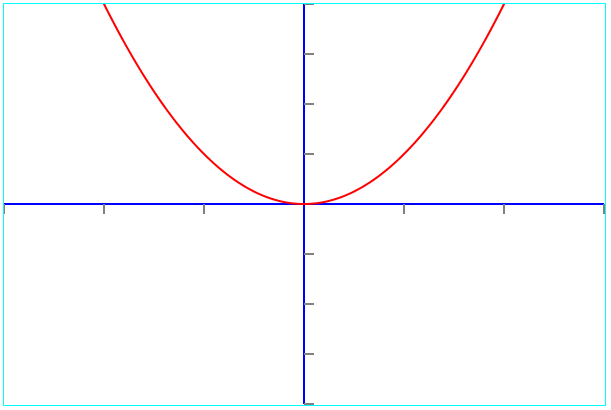# Draw Mathematical Graphs in HTML 5 Canvas

 SEARCH THIS SITE @import url(http://www.google.com/cse/api/branding.css);Custom Search

This tutorial assumes that you are familiar with the drawing on canvas . This tutorial will take through the basic steps in drawing mathematical graphs on an HTML 5 canvas with explanations. Three major steps are described and you can copy the code and try it; see that it should give the same graph shown below each code.

## 1 - Define Scales and Dimensions of Graph and Draw Axes

<!DOCTYPE html>
<html>
<body>
<canvas id="canvasShapes" width="601" height="401" style="border:1px solid #00ffff;">
This browser does not support the HTML5 canvas tag. </canvas>

<script>

var canvas = document.getElementById("canvasShapes");
var ctx = canvas.getContext("2d");

//Define x and y scales
var xScale = 100;
var yScale = 50;

// width and height of canvas in pixels
var width = 600;
var height = 400;

// coordinates of center of canvas in pixels
var xCenter = width/2;
var yCenter = height/2;

// draw axes
ctx.strokeStyle = "blue";
ctx.beginPath();
ctx.lineWidth = 2;

// draw x axis
ctx.moveTo(0,yCenter);
ctx.lineTo(width,yCenter);

// draw y axis
ctx.moveTo(xCenter,0);
ctx.lineTo(xCenter,height);
ctx.stroke();

</script>

</body>
</html>

Explanations

• The statements var xScale = 100; and var yScale = 50; define the scales: 100 pixels along the x axis represents 1 unit and 50 pixels along the y axis represents 1 unit.

• The statements var width = 600; and var height = 400; assign 600 and 400 to the variables width and height respectively.

• The statements var xCenter = width/2; and var yCenter = height/2; define the coordinates of the center of the canvas.

• The statements ctx.moveTo(0,yCenter); and ctx.lineTo(width,yCenter); draw the x axis

• the statements ctx.moveTo(xCenter,0); and ctx.lineTo(xCenter,height); draw the y axis

If you run the above code, you should be able to obtain the following graph..

## 2 - Add Ticks to the Axes

<!DOCTYPE html>
<html>
<body>
<canvas id="canvasShapes" width="601" height="401" style="border:1px solid #00ffff;">
This browser does not support the HTML5 canvas tag. </canvas>
<script>

var canvas = document.getElementById("canvasShapes");
var ctx = canvas.getContext("2d");

//Define x and y scales
var xScale = 100;
var yScale = 50;

// width and height of canvas in pixels
var width = 600;
var height = 400;

// coordinates of center of canvas in pixels
var xCenter = width/2;
var yCenter = height/2;

// draw axes
ctx.strokeStyle = "blue";
ctx.beginPath();
ctx.lineWidth = 2;

// draw x axis
ctx.moveTo(0,yCenter);
ctx.lineTo(width,yCenter);

// draw y axis
ctx.moveTo(xCenter,0);
ctx.lineTo(xCenter,height);
ctx.stroke();

// draw ticks of 1 unit (=100pixels) along x axis
ctx.strokeStyle = "grey";
ctx.beginPath();
ctx.lineWidth = 2;
for (i=1;i<=3;i++)
{
ctx.moveTo(xCenter+i*xScale,200);
ctx.lineTo(xCenter+i*xScale,210);
}
for (i=1;i<=3;i++)
{
ctx.moveTo(xCenter-i*xScale,200);
ctx.lineTo(xCenter-i*xScale,210);
}
ctx.stroke();

// draw ticks of 1 unit (=50pixels) along y axis
ctx.strokeStyle = "grey";
ctx.beginPath();
ctx.lineWidth = 2;
for (i=1;i<=4;i++)
{
ctx.moveTo(xCenter,yCenter+i*yScale);
ctx.lineTo(xCenter+10,yCenter+i*yScale);
}
for (i=1;i<=4;i++)
{
ctx.moveTo(xCenter,yCenter-i*yScale);
ctx.lineTo(xCenter+10,yCenter-i*yScale);
}
ctx.stroke();

</script>
</body>
</html>

Explanations

• The statements // draw ticks of 1 unit (=100pixels) along x axis
ctx.strokeStyle = "grey";
ctx.beginPath();
ctx.lineWidth = 2;
for (i=1;i<=3;i++)
{
ctx.moveTo(xCenter+i*xScale,200);
ctx.lineTo(xCenter+i*xScale,210);
}
for (i=1;i<=3;i++)
{
ctx.moveTo(xCenter-i*xScale,200);
ctx.lineTo(xCenter-i*xScale,210);
}
ctx.stroke();
draw ticks separated by 100 pixels (1 unit) along the x axis.

• The statements // draw ticks of 1 unit (= 50pixels) along y axis
ctx.strokeStyle = "grey";
ctx.beginPath();
ctx.lineWidth = 2;
for (i=1;i<=4;i++)
{
ctx.moveTo(xCenter,yCenter+i*yScale);
ctx.lineTo(xCenter+10,yCenter+i*yScale);
}
for (i=1;i<=4;i++)
{
ctx.moveTo(xCenter,yCenter-i*yScale);
ctx.lineTo(xCenter+10,yCenter-i*yScale);
}
ctx.stroke();
draw ticks separated by 50 pixels (1 unit) along the y axis.

If you run the above code, you should be able to obtain the following graph..

## 3 - Evaluate Function and Graph It

<!DOCTYPE html>
<html>
<body>
<canvas id="canvasShapes" width="601" height="401" style="border:1px solid #00ffff;">
This browser does not support the HTML5 canvas tag. </canvas>
<script>

var canvas = document.getElementById("canvasShapes");
var ctx = canvas.getContext("2d");

//Define x and y scales
var xScale = 100;
var yScale = 50;

// width and height of canvas in pixels
var width = 600;
var height = 400;

// coordinates of center of canvas in pixels
var xCenter = width/2;
var yCenter = height/2;

// draw axes
ctx.strokeStyle = "blue";
ctx.beginPath();
ctx.lineWidth = 2;

// draw x axis
ctx.moveTo(0,yCenter);
ctx.lineTo(width,yCenter);

// draw y axis
ctx.moveTo(xCenter,0);
ctx.lineTo(xCenter,height);
ctx.stroke();

// draw divisions of 1 unit (=100pixels) along x axis
ctx.strokeStyle = "grey";
ctx.beginPath();
ctx.lineWidth = 2;
for (i=1;i<=3;i++)
{
ctx.moveTo(xCenter+i*xScale,200);
ctx.lineTo(xCenter+i*xScale,210);
}
for (i=1;i<=3;i++)
{
ctx.moveTo(xCenter-i*xScale,200);
ctx.lineTo(xCenter-i*xScale,210);
}
ctx.stroke();

// draw divisions of 1 unit (=50pixels) along y axis
ctx.strokeStyle = "grey";
ctx.beginPath();
ctx.lineWidth = 2;

for (i=1;i<=4;i++)
{
ctx.moveTo(xCenter,yCenter+i*yScale);
ctx.lineTo(xCenter+10,yCenter+i*yScale);
}
for (i=1;i<=4;i++)
{
ctx.moveTo(xCenter,yCenter-i*yScale);
ctx.lineTo(xCenter+10,yCenter-i*yScale);
}
ctx.stroke();

// graph function
ctx.translate(xCenter,yCenter);
var y=[ ];
var x=[ ];
var xx;

// calculate function y = x^2 from x = -3 to x = 3
for (i=0;i<=width+1;i++)
{
x[i] =xCenter-i;
xx=x[i]/xScale;
y[i] = -yScale*Math.pow(xx,2);
}
ctx.strokeStyle = "red";
ctx.beginPath();
ctx.lineWidth = 2;
for (i=0;i<=600;i++)
{
ctx.moveTo(x[i],y[i]);
ctx.lineTo(x[i+1],y[i+1]);
}

ctx.stroke();

</script>
</body>
</html>

Explanations

• The statement ctx.translate(xCenter,yCenter); position the graph in the center of the canvas.

• The statements var y=[ ]; var x=[ ]; and define array x and y for the value of the variable x and the value of the function y = f(x).

• The statements for (i=0;i<=width+1;i++)
{
x[i] =xCenter-i;
xx=x[i]/xScale;
y[i] = -yScale*Math.pow(xx,2);
}
calculate the function and scale the x and y coordinates to be used for graphing.

• The statements for (i=0;i<=600;i++)
{
ctx.moveTo(x[i],y[i]);
ctx.lineTo(x[i+1],y[i+1]);
}
plot the graph of the function as small segments.

If you run the above code, you should be able to obtain the following graph..